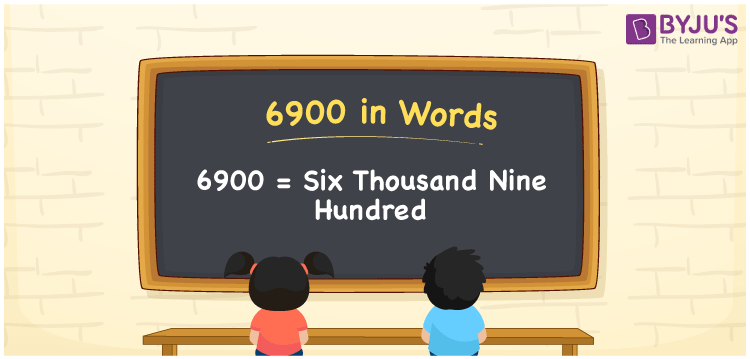# 6900 in Words

6900 in words is written as “Six Thousand Nine Hundred”. In Maths, 6900 is a cardinal number that expresses a quantity. To write a cheque for Rs.6900, we can write in words as Rupees Six Thousand Nine Hundred only. Learn more about Numbers In Words and writing the number names in English at BYJU’S.

 6900 in Words Six Thousand Nine Hundred Six Thousand Nine Hundred in Numbers 6900

## 6900 in English Words

To make it easy for children to learn the names of the numbers we have represented the chart below. It shows the name of 6900 in English words.## How to Write 6900 in Words?

The number 6900 in words can be written using a place value system, where we can identify the position of each digit of the original number. Since 6900 is a four-digit number, thus,

 Thousands Hundreds Tens Ones 6 9 0 0

From the above table,

6 → Thousands

9 → Hundreds

0 → Tens

0 → Ones

Hence, when we read the number from right to left, it is given by Six Thousand Nine Hundred.

### Expanded Form of 6900

We can write the expanded form as:

6 x Thousand + 9 × Hundred + 0 × Ten + 0 × One

= 6 x 1000 + 9 × 100 + 0 × 10 + 0 × 1

= 6000 + 900 + 0 + 0

= Six Thousand Nine Hundred

6900 is a whole number that is succeeded by 6899 and preceded by 6901. Learn more about the number 6900 below:

• 6900 in Words – Six Thousand Nine Hundred
• Is 6900 an odd number? – No
• Is 6900 an even number? – Yes
• Is 6900 a perfect square number? – No
• Is 6900 a perfect cube number? – No
• Is 6900 a prime number? – No
• Is 6900 a composite number? – Yes

## Frequently Asked Questions on 6900 in words

Q1

### What is 6900 in words?

6900 in words is given by Six Thousand Nine Hundred.
Q2

### What is the rule to write 6900 in words?

To write the 6900 in words, we should use place value rule, where the position of each digit helps in determining the name of the number.

6 → Thousands

9 → Hundreds

0 → Tens

0 → Ones

Hence, the number 6900 is read as Six Thousand Nine Hundred.

Q3

### What is the value of 6900 + 100 in words?

6900 + 100 = 7000, i.e., Seven thousand in words.